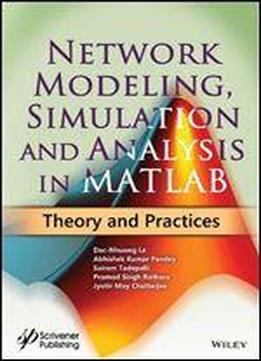# Network Modeling, Simulation And Analysis In Matlab: Theory And Practices by Dac-Nhuong Le / 2019 / English / PDF

The purpose of this book is first to study MATLAB programming concepts, then the basic concepts of modeling and simulation analysis, particularly focus on digital communication simulation. The book will cover the topics practically to describe network routing simulation using MATLAB tool. It will cover the dimensions like Wireless network and WSN simulation using MATLAB, then depict the modeling and simulation of vehicles power network in detail along with considering different case studies. Key features of the book include: Discusses different basics and advanced methodology with their fundamental concepts of exploration and exploitation in NETWORK SIMULATION. Elaborates practice questions and simulations in MATLAB Student-friendly and Concise Useful for UG and PG level research scholar Aimed at Practical approach for network simulation with more programs with step by step comments. Based on the Latest technologies, coverage of wireless simulation and WSN concepts and implementations

views: 283